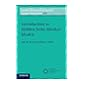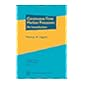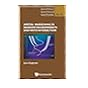Normal view MARC view ISBD view

# Continuous time Markov processes : an introduction / Thomas M. Liggett.

Material type:TextSeries: Graduate studies in mathematics: v. 113.Publisher: Providence, R.I. : American Mathematical Society, c2010Description: xii, 271 p., USD 55.00 27 cm.ISBN: 9780821849491 (alk. paper); 0821849492 (alk. paper).Subject(s): Markov processes | Stochastic integralsDDC classification: 519.2/33
Contents:
One-dimensional Brownian motion -- Continuous time Markov chains -- Feller processes -- Interacting particle systems -- Stochastic integration -- Multi-dimensional Brownian motion and the Dirichlet problem.
Summary: "Markov processes are among the most important stochastic processes for both theory and applications. This book develops the general theory of these processes and applies this theory to various special examples. The initial chapter is devoted to the most important classical example--one-dimensional Brownian motion. This, together with a chapter on continuous time Markov chains, provides the motivation for the general setup based on semigroups and generators. Chapters on stochastic calculus and probabilistic potential theory give an introduction to some of the key areas of application of Brownian motion and its relatives. A chapter on interacting particle systems treats a more recently developed class of Markov processes that have as their origin problems in physics and biology."--Publisher's description.
Item type Current location Call number Status Date due Barcode Item holds
Book Chennai Mathematical Institute
General Stacks
519.233 LIG (Browse shelf) Available 9107
Total holds: 0
##### Browsing Chennai Mathematical Institute Shelves , Shelving location: General Stacks Close shelf browser
 No cover image available No cover image availableNo cover image available No cover image available519.233 DYN Markov Processes/ Vol. 2. 519.233 HOE Introduction to hidden semi-Markov models / 519.233 HOE Introduction to hidden semi-Markov models / 519.233 LIG Continuous time Markov processes : an introduction / 519.233 MAN Analytical treatment of one-dimensional Markov processes. 519.234 ENG Spatial branching in random environments and with interaction / 519.236 SHM Spatially independent Martingales, intersections, and applications /

Includes bibliographical references and index.

One-dimensional Brownian motion -- Continuous time Markov chains -- Feller processes -- Interacting particle systems -- Stochastic integration -- Multi-dimensional Brownian motion and the Dirichlet problem.

"Markov processes are among the most important stochastic processes for both theory and applications. This book develops the general theory of these processes and applies this theory to various special examples. The initial chapter is devoted to the most important classical example--one-dimensional Brownian motion. This, together with a chapter on continuous time Markov chains, provides the motivation for the general setup based on semigroups and generators. Chapters on stochastic calculus and probabilistic potential theory give an introduction to some of the key areas of application of Brownian motion and its relatives. A chapter on interacting particle systems treats a more recently developed class of Markov processes that have as their origin problems in physics and biology."--Publisher's description.